Tamilnadu State Board New Syllabus Samacheer Kalvi 8th Maths Guide Pdf Chapter 3 Algebra Ex 3.2 Text Book Back Questions and Answers, Notes.

## Tamilnadu Samacheer Kalvi 8th Maths Solutions Chapter 3 Algebra Ex 3.2

Question 1.
Fill in the blanks
(i)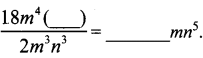$$\frac{18 m^{4}\left(n^{8}\right)}{2 m^{(3)} n^{3}}$$ = 9 mn5(ii)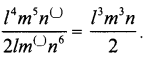$$\frac{l^{4} m^{5} n^{(7)}}{2 l m^{(3)} n^{6}}=\frac{l^{3} m^{2} n}{2}$$

(iii)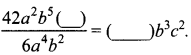$$\frac{42 a^{4} b^{5}\left(c^{2}\right)}{6(a)^{4}(b)^{2}}$$ = (7)b3c2

Question 2.
Say True or False
(i) 8x3y ÷ 4x2 = 2xy
True(ii) 7ab3 ÷ 14 ab = 2b2
False

Question 3.
Divide
(i) 27 y3 by 3y
(ii) x3 y2 by x2y
(iii) 45x3 y2 z4 by (-15 xyz)
(iv) (3xy)2 by 9xy
(i) 27 y3 by 3y
$$\frac{27 y^{3}}{3 y}$$ = $$\frac{27}{3} y^{3-1}$$ = 9y2

(ii) x3 y2 by x2y
$$\frac{x^{3} y^{2}}{x^{2} y}$$ = x3-2 y2-1 = x1 y1 = xy

(iii) 45x3 y2 z4 by (-15 xyz)
$$\frac{45 x^{3} y^{2} z^{4}}{-15 x y z}$$ = $$\frac{45}{-15}$$ x3-2 y2-1 y4-1 z4-1 = -3x2 yz3(iv) (3xy)2 by 9xy
$$\frac{(3 x y)^{2}}{3 \times(3 x y)}$$ = $$\frac{(3 x y)^{2}}{3 \times(3 x y)}$$ = $$\frac{1}{3}$$ (3xy)2-1 = $$\frac{1}{3}$$ 3xy = xy

Question 4.
Simplify
(i) $$\frac{3 m^{2}}{m}+\frac{2 m^{4}}{m^{3}}$$
(ii) $$\frac{14 p^{5} q^{3}}{2 p^{2} q} \frac{12 p^{3} q^{4}}{3 q^{2}}$$
(i) $$\frac{3 m^{2}}{m}+\frac{2 m^{4}}{m^{3}}$$
$$\frac{3 m^{2}}{m}+\frac{2 m^{4}}{m^{3}}$$ = 3m2-1 + 2m4-3
= 3m + 2m
= (3 + 2) m
= 5m

(ii) $$\frac{14 p^{5} q^{3}}{2 p^{2} q} \frac{12 p^{3} q^{4}}{3 q^{2}}$$
$$\frac{14 p^{5} q^{3}}{2 p^{2} q} \frac{12 p^{3} q^{4}}{3 q^{2}}$$ = $$\frac{14}{2}$$p5-2q3-1 – $$\frac{12}{3}$$ p3q4-3
= 7p3q2 – 4p3qQuestion 5.
Divide:
(i) 32y2 – 8yz by 2y
(ii) (4m2n3 + 16m4 n2 – mn) by 2mn
(iii) 5xy2 – 18x2y3 + 6xy by 6xy
(iv) 81(p4 q2 r3 + 2p3q3 r2 – 5p2q2r2) by (3pqr)2
(i) 32y2 – 8yz by 2y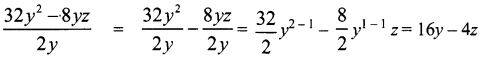(ii) (4m2n3 + 16m4 n2 – mn) by 2mn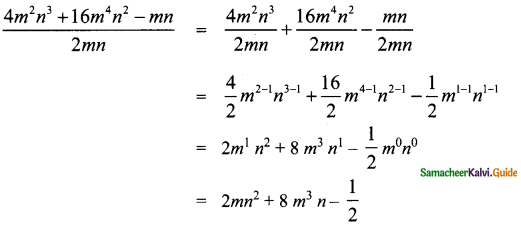(iii) 5xy2 – 18x2y3 + 6xy by 6xy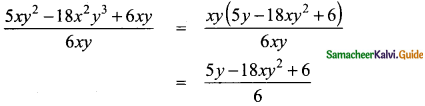(iv) 81(p4 q2 r3 + 2p3q3 r2 – 5p2q2r2) by (3pqr)2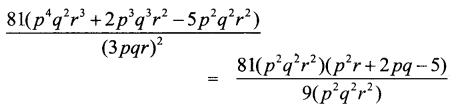= $$\frac{81}{9}$$(p2q2r2)1-1 (p2r + 2pq – 5)
= 9(p2r + 2pq – 5) = 9 p2r + 18pq – 45Question 6.
Identify the errors and correct them.
(i) 7y2 – y2 + 3y2 = 10y2
7y2 – y2 + 3y2 = 10y2 = (7 – 1 + 3)y2
= (6 + 3)y2
= 9y2

(ii) 6xy + 3xy = 9x2y2
6xy + 3xy = (6 + 3) xy
= 9 xy

(iii) m(4m – 3) = 4m2 – 3
m(4m – 3) = m(4m) + m(-3)
= 4m2 – 3m(iv) (4n)2 – 2n + 3 = 4n2 – 2n + 3
(4n)2 – 2n + 3 = 16n2 – 2n + 3

(v) (x – 2)(x + 3) = x2 – 6
(x – 2)(x + 3) = x(x + 3) – 2 (x + 3)
= x(x) + (x) × 3 + (-2) (x) + (-2) (3)
= x2 + 3x – 2x – 6
= x2 + x – 6

(vi) -3p2 + 4p – 7 = -(3p2 + 4p – 7)
-3p2 + 4p – 7 = -(3p2 – 4p + 7)

Question 7.
Statement A: If 24p2q is divided by 3pq, then the quotient is 8p.
Statement B: Simplification of $$\frac{(5 x+5)}{5}$$ is 5x.
(i) Both A and B are true
(ii) A is true but B is false
(iii) A is false but B is true
(iv) Both A and B are false
(ii) A is true but B is false
Hint: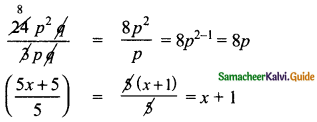Question 8.
Statement A: 4x2 + 3x – 2 = 2(2x2 + $$\frac{3 x}{2}$$ – 1)
Statement B: (2m – 5) – (5 – 2m) = (2m – 5) + (2m – 5)
(i) Both A and B are true
(ii) A is true but B is false
(iii) A is false but B is true
(iv) Both A and B are false